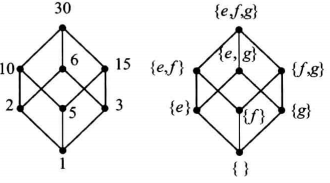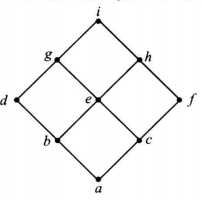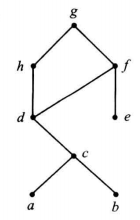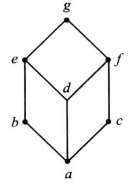## 基本概念

$\left| G \right|$ 记号 ：表示集合 $G$ 中元素的个数。

Dom：是定义域记号。

Ran：是值域记号。

1--->2
3----↑


a 的入度 ：就是有向图上指向 $a$ 的箭头数。

a 的出度 ：就是有向图上离开 $a$ 的箭头数。

a 到 b 的路径 ：是有向图上从 $a$$b 加上途径元素的一条有限序列 a, x_1, x_2, \cdots , x_{n-1}, b。算上 a, b 一共是 n+1 个元素。长度定为 n 自反关系 ：是指对每个元素，都存在自己指向自己的关系对，的关系。 对称关系 ：是指任意两个元素 a,b，如果 a 指向 b，一定有 b 指向 a，的关系。（如果 a R b$$b R a$）。其关系矩阵是对称的。

p 决定的 A 上的等价关系

LUB$a$ 是最小上界，就是没有比 $a$ 更小的上界 $a'$

GLB$a$ 是最大下界，就是没有比 $a$ 更大的下界 $a'$

：如果一个偏序集，其任意两个元素构成的子集都有 LUB 和 GLB，那这个偏序集是一个格。$a \vee b$ 表示 $\operatorname {LUB}({a,b})$$a \wedge b 表示 \operatorname {GLB}({a,b}) 子格 ：设 <S, ∧, ∨> 构成格，L 是 S 的非空子集，如果 L 关于格中的运算∨和∧仍然能够构成格，那么 L 就是 S 的子格。这里建议结合子群的概念理解。 同构的格 ：说简单点就是两个格哈斯图一样。 分配格 ：如果对该格有 a∧(b∨c) = (a∧c)∨(a∧b), 即满足分配率的话，就是分配格 封闭的二元运算 ：指所有二元运算的结果仍然在该运算相关的集合内。 半群 （封闭 + 结合） 半群就是非空集合 S 以及一个定义在 S 上的可结合的二元运算 * ，用 (S，*) 表示半群，省略形式 S 单位元（幺元）：若存在 e，使得 a * e = e * a = a，那么 e 是单位元。（其中，a, e \in G$$G$ 是这个半群的集合）。满足 $a * e = e$ 的是 左幺元，同理可知右幺元。

XX （半）群 ：具有 XX 性质的（半）群。比如：

（全部可逆 + 幺半群） 群就是每个元都有逆元的幺半群。群的单位元和逆元都是唯一的。并且群满足可消去律。

$n$ 元置换 ：被置换的集合元素是 $n$ 个，这个置换函数是 $n$ 元置换。

|G/H| 表示 $H 在$G$中的指数，记作$[G:H]$拉格朗日定理 有限群$G$$H < G，则 |G| = [G:H] \cdot |H| 商群 ：就是商群与同余关系构成的群。为了让关系 R 满足同余的条件，需要能从 a_1 R b_1, a_2 R b_2 推出 a_1 a_2 R b_1 b_2，也即 a_2^{-1} a_1^{-1} b_1 b_2 \in H，也即 g^{-1} h g \in H$$g \in G, h \in H$同余关系的充要条件$g^{-1} h g \in H$$g \in G, h \in H 正规子群（normal subgroup, invariant subgroup） 满足上述同余条件的子群。即：G 为群，H < G，如果满足，\forall g \in G, h \in H$$ghg^{-1} \in H$$H$$G$的正规子群。 平凡子群 ：对 G，平凡子群是自己和幺元（$G$$H = {e}），它一定是正规的。 交换群子群皆正规 群的积 G = G_1 \times G_2 通过 (a_1, b_1) (a_2, b_2) = (a_1 a_2, b_1 b_2) 定义。 群同态的性质：若 f:G_1 \to G_2 是群的同态，则 f (G_1) < f (G_2) 核（kernel）：如果 g \in G_1 使得同态 f:G_1 \to G_2 映射的结果是单位元，那么 g 是核中元素，所有满足条件的 g$$f$的核，记作$\operatorname {Ker} f$核不但是第一个群的子群，而且是正规的：$\operatorname {Ker} f \triangleleft G_1$。原因在于$\forall a, b \in \operatorname {Ker} f, f (ab^{-1}) = f (a) f (b)^{-1} = e_2 e_2^{-1} = e_2$，这说明$\operatorname {Ker} f \in G_1$$f (ghg^{-1}) 在核中，所以满足正规子群的定义。 自然同态 f:G \to G/H 得到核的方法 ：若 H \triangleleft G$$f$是自然同态，则$\operatorname {Ker} f = H$同态基本定理$f: G_1 \to G_2$是满同态，则$G_1/ \operatorname {Ker} f \simeq G_2$同态是单同态的充要条件 ：同态的核只有单位元。$\operatorname {Ker} f = \{e_1\}$B^m：若有集合$B = \{0,1\}$，则$(B, \operatorname {xor})$是一个群。$B^m = B \times B \times B \times \cdots \times B$$\operatorname {xor} 运算下也是群。 编码函数 ：若有 n>m，单射函数 e: B^m \to B^n 是一个 (m,n) 编码函数。 代码字 ：编码前的任何一个信息位序列编码后的序列。即 如果 b \in B^m，则 e (b)$$b$的代码字。 ：就是二进制序列中$1$的个数，记作$|x|$奇 / 偶校验（Parity Check）：就是通过向二进制序列追加一个位，使得编码后序列的权为奇 / 偶数。 Hamming 距离 ：就是两个二进制序列异或之后序列的权$|x \operatorname {xor} y |$最短距离 ：就是编码后不是有很多可能的序列嘛，这些序列之间的距离的最小值，就是最短距离。 定理 11.1.2：编码函数能检测的最多错误数量，是最短距离减一。 群码 ：如果$e$是群码，表明所有代码字的集合是$B^n$的一个子群。 定理 11.1.3：群码函数的最短距离是非零代码字的最小权。 奇偶校验矩阵 ：描述编码后的信息序列之间的线性关系的矩阵。 译码函数 ：一个满射函数，当没有噪声时，输入编码后的信息，输出原来的信息。 ：若半群（乘法封闭 + 结合）满足加法交换，且关于加法或乘法分配，则是环。 ## 基本题型 ### 如何证明偏序关系 根据定义证。略。 ### 如何证明关系等价 证明：自反对称传递。 【例子】 证明模二同余是等价关系。 【分析与解答】 1. 自反证明：根据定义，$a \equiv a (\mod 2)$成立，因此是自反的。 2. 对称证明：若$a \equiv b (\mod 2)$，则$a \equiv r (\mod 2)$$b \equiv r (\mod 2)，则 b \equiv a (\mod 2) 3. 传递证明：若 a \equiv b (\mod 2)$$b \equiv c (\mod 2)$，则$a \equiv r (\mod 2)$$b \equiv r (\mod 2)$$c \equiv r (\mod 2)$，则$a \equiv c (\mod 2)$### 如何证明关系同构 【例子】 39．设$A=\{1，2，3，5，6，10，15，30\}$，考虑 A 上的整除偏序 ≤，即定义 a≤b 为 a|b。设$A'=P (S)$是有偏序∈的偏序集，这里$S= \{e，f，g\}$。证明:$(A，≤)$$(A'，∈) 是同构的。 【分析与解答】 这里 P 是幂集，展开来 P (S) = \{\{\}, \{e\}, \{f\}, \{g\}, \{e, f\} , \{e, g\}, \{f,g\}, \{e,f,g\}\}。再把 A 上的整除关系画出来，就能发现同构了。据此可以定义一一对应的函数，从而证明是同构。 ### 如何证明群 / 半群同构 证明同构就是证明通过一个映射能够让两个半群完全对应起来。所以 1. 首先就要构造 f 2. 然后证明一一对应（满射，单射分别证明）， 3. 最后证明运算规则也一样（f (ab) = f (a) f (b) 同构关系是对称的，所以你证明 a 同构于 b 或是相反，都可以。 【例子】 设 T 是所有偶数的集合，证明半群 (Z,+)$$(T,+)$是同构的。 【分析与解答】 1. 首先构造$f: Z\to T$，令 a' =$f (a) = 2a, a \in Z$2. 证明单射：假设不是单射，有$f (a_1) = f (a_2), a_1 \neq a_2$, 则$2a_1 = 2a_2$，根据左消去律$a_1 = a_2$，与假设矛盾，所以是单射哒。 3. 证明满射：就是要证明每个$b \in T$都存在$a$，使得$f (a) = b$成立。这么来：取$a = b/2$，根据偶数的性质，则$b/2 \in Z$，则$f (a) = f (b/2) = b$成立。 4. 最后，$f (a+b) = (a+b)/2 = a/2 + b / 2 = f (a)+f (b)$，此证。 ### 从哈塞图判断是不是格不是，观察$\{e, a\}, \{e, b\}, \{a, b\}$，发现每组的两个之间不能比较偏序关系。推荐阅读： https://zhuanlan.zhihu.com/p/24338970 一般来说，出现交叉和悬空都不是格。 【分析与解答】 ### 如何确定划分$A/R$步骤 1: 选 A 中任意一个元素，计算等价类 R (a)。 步骤 2: 如果 R (a)≠A，选一个不包含在 R (a) 中的元素 b，计算等价类 R (b)。 步骤 3: 如果 A 不是上面两步计算出的等价类的并集，那么，选择 A 中的一个元素 x，它不是前面任何等价类中的元素，计算 R (x)。 步骤 4: 重复步骤 3，直到 A 中的所有元素都包含在计算出的等价类中。如果 A 是可数的，那么该过程可能继续无限次。在这种情况下，可继续到一个模型出现，使得能对所有等价类进行描述或者给出一个公式为止。 【例子】$A = \{1,2,3,4\}, R = \{( 1,1) , (1,2), (2,1), (2,2), (3,4), (4,3), (3,3), (4,4)\}$确定$A/R$【分析与解答】 画个图，可以发现$1,2$$3，4 两组是不连着的，所以 A/R = \{\{1,2\}, \{3,4\} ### 如何求关系的补、并、交、逆 例 1 设 A=\{1,2,3,4\}, B=\{a, b, c\}, 且设 R=\{(1, \quad a), \quad(1, b), \quad(2, b), \quad(2, c), \quad(3, b), \quad(4,a) \}, \quad S=\{(1, b),(2, c),(3, b),(4, b)\} 计算: (a) \bar{R} (b) R \cap S (c) R \cup S; (d) R^{-1} 【分析与解答】 补就是把矩阵每个元素进行非运算。交就是与运算，并就是或运算，逆就是转置。 ### 如何用 Warshall 算法求传递闭包 [求传递闭包的 Warshall 算法详解] ### 根据关系的描述画出哈斯图 【例子】 A=\{1,2,3,4\}, R=\{(1,1),(1,2),(2,2),(2,4),(1,3),(3,3),(3,4),(1,4),(4,4)\}. 【分析与解答】  4 / \ 2 3 \ / 1  ### 如何证明是子格 【例子】 证明：全序偏序集的子集是一个子格。 【分析与解答】 根据全序偏序集的定义，对任意 x, y 于其中，有 x \leqslant y$$y \leqslant x$成立。如果$x \leqslant y$，则$x \wedge y$（x，y 的 GLB 最大下界）是$x$$x \vee y = y，反之亦然。所以命题真。 ### 如何证明是子群 老实证法：证明子集（一般是题给条件或者显然的）、满足群性质（运算封闭、可结合、幺元、全部可逆）。 子群 ：假设 G 是群，H 是它的一个非空子集，若 H$$G$的运算构成群，那么$H$就是$G$的子群。记作$H < G$下面先证明$\forall a, b \in H, ab^{-1} \in H$（其中$H$$G 的非空子集） 可以推出 H < G 首先，若 a = b，则 aa^{-1} \in H，也即 e \in H。（这是根据幺元的性质） e \in H, b \in H 可以推出 b^{-1} \in H。（这是根据群的性质，即所有元素都可逆） a \in H, b^{-1} \in H，可以推出 a (b^{-1})^{-1} \in H（这是根据题给条件，其中 b 换成了 b^{-1}）。 上面证明了运算封闭。 由于运算封闭，H 所有的运算连同被运算的 a,b 都是属于大的集合 G，而 G 是群所以运算结合，所以对于 H，结合律也是满足的。 至于幺元和逆，上面证明运算封闭的时候已经证明了 e \in H，且 b^{-1} \in H 这就给了我们一个重要的证法： 通过证明 \forall a, b \in H, ab^{-1} \in H 来证明 H$$G$的子群 下面证明有限子集运算封闭就构成子群。 由于运算封闭，所以小集合$H$继承大集合$G$的结合律和消去律，并且$H$是有限半群，所以它是群（有限半群满足左右消去律，那它就是群）。 判定子群的基本方法就是证明$a^{-1} b$运算结果封闭。 ### 证明子群之交仍是子群$\forall a, b \in H_1 \cap H_2$，只需证明$ab^{-1} \in \in H_1 \cap H_2e \in H_1 \wedge e \in H_2$，所以$e \in H_1 \cap H_2$，这也说明交集不是空集。 由于$H_1< G$$H_2 < G，所以 ab^{-1} \in H_1 \wedge ab^{-1} \in H_2 这就说明 ab^{-1} \in H_1 \cap H_2，从而得证。 【例子】 考虑 P_1 \subseteq P_2，则 <P_1, +>，是 <P_2, +> 的子群。 ### 如何证明二元运算是幂等的？ 幂等：其实就是自反。比如 a 满足 a = a*a$$*$运算是幂等的。 【例子】 证明或者反证$Z^+$上的$a*b = \operatorname {GCD}(a,b)$具有幂等性质。 【分析与解答】 即证$a = \operatorname {GCD}(a, a)$对于所有$a \in Z^+$成立。由于$a /a = 1$余数是$0$，所以$\operatorname {GCD}(a,a) = a$，所以是幂等的。 ### 为什么陪集是等价类？ 首先明白等价类是啥，如果$G$上有等价关系$R$（自反对称传递），那么和$g \in G$等价的所有所有元素构成的集合是等价类$[g]$我们前面提到过，判定子群的基本方法就是证明$a^{-1} b$运算结果封闭。 （其中$a, b \in H, H < G$）。 第一，我们先定义关系 R 通过$a^{-1} b$我们可以建立关系$\mathbf {R}$：所有满足关系$\mathbf {R}$$a,b，都意味着 a^{-1} b \in H 第二，我们证明关系 R 是等价关系 我们先说明 R 是等价关系。自反：a^{-1} a = e \in H，是满足的。对称：a^{-1} b = ((a^{-1} a)^{-1})^{-1} = (b^{-1} a)^{-1} \in H，也满足。传递：(a^{-1} b)(b^{-1} c) = a^{-1} c \in H，依旧满足。所以 \mathbf {R} 是等价关系。 第三，说明 gH 是等价类 要说明 g$$H$的陪集就是等价类，就是说明$gH$中任意两个元素等价。也就是要说明$ga \mathbf {R} gb$，也就是要说明$(ga)^{-1} gb \in H(ga)^{-1} gb = a^{-1} g^{-1} g b = a^{-1} (g^{-1} g) b = a^{-1} b \in H$。所以 陪集中任意两个元素之间都存在 R 这个等价关系，所以$g$$H 的陪集就是等价类 [g]。所有陪集 (g_1H, g_2H, \cdots, g_nH) 就是等价类的集合 —— 构成了 G 的划分（等价类不可能相交，如果重叠就会归为同一个等价类。也不会遗漏，因为对于 G 中每个元素，我们都得到了它的等价类）。 这体现了陪集的一个性质：可以按照某关系将 G 刚好进行划分。 ### 如何证明同态？ 首先想想什么是同态，同构的条件放宽点，能搞出个映射就 OK。 【例子】 证明：函数 f (x)=|x| 是从非零实数群 G 到正实数群 G' 的一个同态，其中群 G$$G'$中的运算是乘法。$f(ab) = |ab| = |a||b| = f(a)f(b)$所以是个同态。 其实还可以玩出点新花样（李云龙.jpg)，证明是满同态： 我们证明它是一个满射，并满足$f (ab) = f (a) f (b)$满射证明：设$b \in G'$，可知$b > 0$，可知$|b| = |-b| = b$，即存在$f (b) = f (-b) = |b|$，所以是满射。 所以是满同态。 【例子】 33．设 G 是一个群。证明：由$f (a)=a^2$定义的函数$f:G→G$是一个同态当且仅当$G$是阿贝尔群。 【分析与解答】 必要性：$f(ab) = (ab)^2 = b^2 a^2 = bbaaf(a) f(b) = a^2 b^2 = aabb$假设$f (ab) = f (a) f (b)$，则$b (ba) a = a (ab) b$（群都满足结合律）推出$b^{-1} a (ab) = (ba) ab^{-1}(ab^{-1})^{-1}(ab) = (ba)(ab^{-1})$,$(ab) = (ba)(ab^{-1})(ab^{-1})^{-1}$,$ab = ba$成立。就是交换律。 充分性：反过来就是了。 ### 如何证明同构 要素：双射，$f (ab) = f (a) f (b)$【例子】 35．设 G 是一个群，a 是 G 中的一个固定元素。证明：对于$x \in G$，由$f (x)=axa^{-1}$定义的函数$f_a:G→G$是一个同构。 【分析与解答】$f (x) f (y) = axa^{-1} aya^{-1} = axy^{-1} a = f (xy)$。所以运算法则是一致的。然后证明双射吧。 单射：即证明不存在不同的$x, y$s.t.$f (x) = f (y)$. 假设$f (x) = f (y)$，则$axa^{-1} = aya^{-1} \to x = y$得证。 满射：即对于所有$y \in G$(destination)，都有$x \in G$(source) s.t.$f (x) = y$。解$f (x) = axa^{-1} = y$，可得$x = a^{-1} ya$，需要证明$a^{-1} ya \in G$.$f (a^{-1} ya) = y \in G$，所以$a^{-1} ya \in G$，此证。 单射：任取$x \in G$，由于$G$是群，所有元素均可逆，所以$x^{-1} \in G$，令$y = axa^{-1}$，则$y^{-1} = a^{-1} xa^{1}$，则$ay^{-1} a^{-1} = x^{-1} \in G$### 如何证明正规子群 下列三个条件等价： 1.$H \triangleleft G$2. 左右陪集相等：$gH = Hg, \forall g \in G$3.$\forall g_1, g_2 \in G, g_1H \cdot g_2H = g_1g_2H$如果$H < G$，关系$R$等价并且同余，那么$H \triangleleft G$### 其它 【例子】 对于$n$元素集合，可构成多少个自指关系？ 【分析与解答】 首先我们有前提：$n$个元素构成的任何集合都是$n\times n $的子集。 ![typora\20201103212157_4685cd0422f887f3d2934f78f475a8c0.png] 构成自指$R$必须包含对角线上的元素，然后剩下的部分可以任意抽取，而剩下的部分有$n^2 - n$个元素，可以构成$2^{n (n-1)}$个子集。 因此答案是$1 \cdot 2^{n (n-1)}$【例子】 Let A be a set with n elements. How many commutative binary operations can be defined on A? 【分析与解答】 构成交换要求运算矩阵对称，$n^{n^2}\$

: https://www.pluvet.com/2020/12/27 / 求传递闭包的 - warshall - 算法详解 / : https://pluvet-1251765364.cos.ap-chengdu.myqcloud.com/typora%5C20201103212157_4685cd0422f887f3d2934f78f475a8c0.png